# GSEB Solutions Class 7 Maths Chapter 1 Integers Ex 1.1

Gujarat Board GSEB Textbook Solutions Class 7 Maths Chapter 1 Integers Ex 1.1 Textbook Questions and Answers.

## Gujarat Board Textbook Solutions Class 7 Maths Chapter 1 Integers Ex 1.1Question 1.
Following number line shows the temperature in degree Celsius (°C) at different places on a particular day.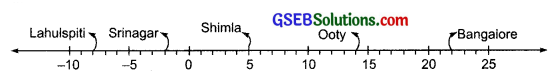(a) Observe this number line and write the temperature of the places marked on it.
(b) What is the temperature difference between the hottest and the coldest places among the above?
(c) What is the temperature difference between Lahulspiti and Srinagar?
(d) Can we say temperature of Srinagar and Shimla taken together is less than the temperature at Shimla? Is it also less than the temperature at Srinagar?
Solution:
(a)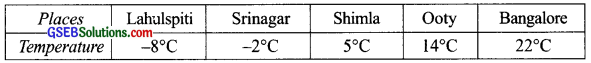(b) Here, the hottest place is Bangalore (22°C) and the coldest place is Lahulspiti (- 8°C).
∴ Temperature difference between the hottest and the coldest places
= 22°C – (-8°C)
= 22°C + 8°C
= 30°C

(c) ∵ Temperature at Lahulspiti = – 8°C and temperature at Srinagar = – 2°C
∴ Required difference = – 2°C – ( – 8°C) [∵ (- 2) > (- 8)]
= – 2°C + 8°C = 6°C

(d) ∵ The sum of the temperatures of Srinagar and Shimla
= (- 2°C) + 5°C = 3°C and 3°C < 5°C Yes, sum of temperatures at Srinagar and Shimla is less than the temperature at Shimla. Temperature of Srinagar = – 2°C Also, 3°C > – 2°C
No, the temperature at Srinagar and Shimla is not less than the temperature at Srinagar.Question 2.
In a quiz, positive marks are given for correct answers and negative marks are given for incorrect answers. If Jack’s scores in five successive rounds were 25, -5, -10, 15 and 10, what was his total at the end?
Solution:
∵ The score of 1st round = 25
The score of 2nd round = – 5
The score of 3rd round = – 10
The score of 4th round = 15
The score of 5th round = 10
∴ Total score = 25 + (- 5) + (- 10) + 15 + 10
= 50 – 15
= 35
Hence, total score of Jack = 35

Question 3.
At Srinagar, temperature was – 5°C on Monday and then it dropped by 2°C on Tuesday. What was the temperature of Srinagar on Tuesday? On Wednesday, it rose by 4°C, then what was the temperature on this day?
Solution:
At Srinagar, temperature on Monday = – 5°C
As the temperature falls by 2°C
∴ Temperature on Tuesday = – 5°C + (- 2°C)
= – 7°C
The temperature increased by 4°C on Wednesday
∴ Temperature on Wednesday = – 7°C + 4°C
= – 3°C

Question 4.
A plane is flying at the height of 5000 m above the sea level. At a particular point, it is exactly above a submarine floating 1200 m below the sea level. What is the vertical 5000 distance between them?
Solution:
Since, the sea level is at 0 m and the plane is 5000 m above the sea level.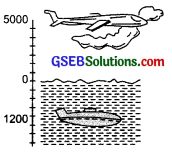∴ Distance between plane and the sea level = 5000 m
Also, the submarine is 1200 m below the sea level.
∴ Distance between submarine and the sea level = 1200 m
∴ Distance between the plane and the submarine = 5000 m + 1200 m
= 6200 mQuestion 5.
Mohan deposits ₹ 2000 in his bank account and withdraws ₹ 1642 from it, the next day. If withdrawal of amount from the account is represented by a negative integer, then how will you represent the amount deposited? Find the balance in Mohan s account after the withdrawal.
Solution:
Since, withdrawing money from the bank is opposite to depositing money to the bank. Therefore, the amount deposited is represented by a positive integer.
Now, amount deposited = + ₹ 2000
Amount withdrawn = – ₹ 1642
∴ Balance, after withdrawal = ₹ (2000 – 1642)
= ₹ 358

Question 6.
Rita goes 20 km towards east from a point A to the point B. From B she moves 30 km towards west along the same road. If the distance towards east is represented by a positive integer, then, how will you represent the distance travelled towards west? By which integer will you represent her final position from A?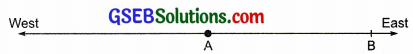Solution:
The directions east and west are opposite to each other.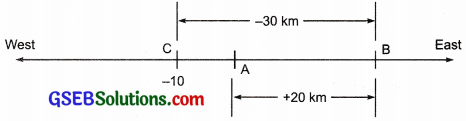∴ If moving towards east is a positive integer, then moving towards west is represented by a negative integer.
Now, distance moved towards east = + 20 km
And, distance moved towards west = – 30 km
Also, (- 30) + (+ 20) = – 10
∴ The final position is represented by – 10.

Question 7.
In a magic square each row, column and diagonal have the same sum. Check which of the following is a magic square.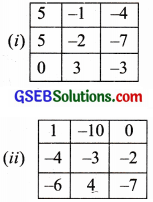Solution:
(i) Magic Square:
Sum of digits along:
1st row = 5 + (- 1) + (- 4) = 5 + (- 5) = 0
2nd row = (- 5) + (- 2) + 7 = – 7+ 7 = 0
3rd row = 0 + 3 + (- 3) = 3 + (- 3) = 0
1st column = 5 + (- 5) + 0 = 5 + (- 5) = 0
2nd column = (- 1) + (- 2) + 3 = (- 3) + 3 = 0
3rd column = (- 4) + 7 + (- 3) = (- 7) + 7 = 0
One diagonal = 5 + (- 2) + (- 3) = 5 – 5 = 0
Other diagonal = 0 + (- 2) + (- 4) = 0 + (- 6)
= – 6
∵- 6 ≠ 0
∴ Square (i) is not a magic square.

(ii) Magic Square:
Sum of the digits along:
1st row = 1 + (- 10) + 0
= 1 – 10 = – 9
2nd row = (- 4) + (- 3) + (- 2)
= (- 7) + (- 2) = – 9
3rd row = (- 6) + 4 + (- 7)
= (- 13) + 4 = – 9
1st column = 1 + (- 4) + (- 6)
= 1 – 10 = – 9
2nd column = (- 10) + (- 3) + 4
= (- 13) + 4 = – 9
3rd column = 0 + (- 2) + (- 7)
= 0 + (- 9) = – 9
One diagonal = 1 + (- 3) + (- 7)
= 1 + (- 10) = – 9
Second diagonal = (- 6) + (- 3) + 0
= (- 9) + 0 = – 9
∵ Each row, column and diagonal have the same sum.
∴ The square (ii) is the magic square.Question 8.
Verify a – (-b) = a + b for the following values of a and b.
(i) a = 21, b = 18
(ii) a = 118, b = 125
(iii) a = 75, b = 84
(iv) a = 28, b = 11
Solution:
(i) Given that: a = 21 and b = 18
∴ L.H.S. = a – (-b)
= 21 – (-18) = 21 + 18 = 39
and, R.H.S. = a + b = 21 + 18 = 39
Since, L.H.S. = R.H.S.
∴ a – (- b) = a + b is verified.

(ii) Given that: a = 118 and b = 125
∴ L.H.S. = a – (-b)
= 118 – (- 125)
= 118 + 125 = 243
and, R.H.S. = a + b
= 118 + 125 = 243
Since, L.H.S. = R.H.S.
∴ a – (- b) = a + b is verified.

(iii) Given that: a = 75 and b = 84
∴ L.H.S. = a – (-b)
= 75 – (- 84) = 75 + 84
= 159
and, R.H.S. = a + b
= 75 + 84 = 159
Since, L.H.S. = R.H.S.
∴ a – (-b) = a + b is verified.

(iv) Given that: a = 28 and b = 11
∴ L.H.S. = a – (- b)
= 28 – (- 11) = 28 + 11
= 39
and R.H.S. = a + b
= 28 + 11 = 39
Since, L.H.S. = R.H.S.
∴ a – (-b) = a + b is verified.

Question 9.
Use the sign of >,< or = in the box to make the statements true.
(a) (- 8) + (- 4) ☐ ( – 8) – (- 4)
(b) (- 3) + 7 – (19) ☐ 15 – 8 + (- 9)
(c) 23 – 41 + 11 ☐ 23 – 41 – 11
(d) 39 + (- 24) – (15) ☐ 36 + (- 52) – (-36)
(e) – 231 + 79 + 51 ☐ – 399 + 159 + 81
Solution:
(a) (- 8) + ( – 4) = – 12
(- 8) – (- 4) = – 8 + 4 = – 4
(- 12) < (- 4)
∴ (- 8) + (- 4)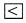(- 8) – (- 4)

(b) (- 3) + 7 – 19 = – 22 + 7 = – 15
15 – 8 + (- 9) = 15 – 17 = – 2
(- 15) < (- 2)
∴ (- 3) + 7 – 1915 – 8 + (- 9)

(c) 23 – 41 + 11 = 34 – 41 = – 7
23 – 41 – 11 = 23 – 52 = – 29
(- 7) > (- 29)
∴ 23 – 41 + 11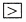23 – 41 – 11

(d) 39 + (- 24) – 15 = 39 + (- 39) = 0
36 + (- 52) – (- 36) = 72 + (- 52) = 20
0 < 20
∴ 39 + (- 24) – 1536 + (- 52) – (-36)

(e) – 231 + 79 + 51 = – 231 + 130 = – 101
– 399 + 159 + 81 = – 399 + 240 = – 159
(- 101) > (- 159)
∴ – 231 + 79 + 51– 399 + 159 + 81Question 10.
A water tank has steps inside it. A monkey is sitting on the topmost step (i.e., the first step). The water level is at the ninth step.
(i) He jumps 3 steps down and then umps back 2 steps up. In how many jumps will he reach the water level?
(ii) After drinking water, he wants to go back. For this, he jumps 4 steps up and then jumps back 2 steps down in every move. In how many jumps will he reach back the top step?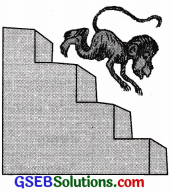(iii) If the number of steps moved down is represented by negative integers and the number of steps moved up by positive integers, represent his moves in part (i) and (ii) by completing the following; (a) – 3 + 2 – … = – 8 (b) 4 – 2 + … = 8. In (a) the sum (- 8) represents going down by eight steps. So what will the sum 8 in (b) represent?
Solution:
(i) The monkey is sitting on the top (i.e., the first step).
∴ The position of monkey after the

• 1st Jump is at fourth step.
• 2nd Jump is at second step.
• 3rd Jump is at fifth step.
• 4th Jump is at third step.
• 5th Jump is at sixth step.
• 6th jump is at fourth step.
• 7th jump is at seventh step.
• 8th jump is at fifth step.
• 9th jump is at eight step.
• 10th jump is at sixth step.

11th jump is at ninth step, which is the water level. Thus, required number of jumps =11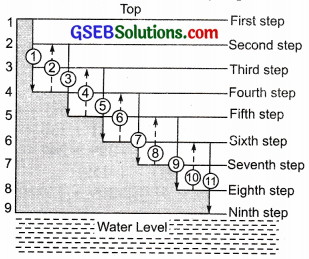(ii) The monkey’s position is at the water level (the ninth step).
∴ The position of monkey after the

• 1st jump is at fifth step.
• 2nd jump is at seventh step.
• 3rd jump is at third step.
• 4th jump is at fifth step.
• 5th jump is at first (top) step.

Thus, the required number of jumps = 5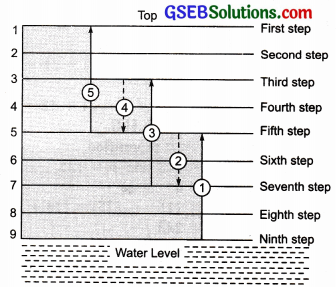(iii) Since, the number of steps moved by the monkey towards the top is taken as positive and the number of steps moved towards the water level is taken as negative.
∴ In case (i) we have: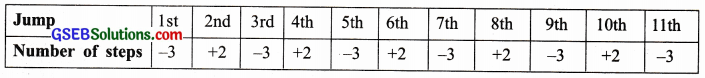∴ (a) Total number of steps = – 3 + 2 – 3 + 2 – 3 + 2 – 3 + 2 – 3 + 2 – 3 = – 8
Again in case (ii), we have: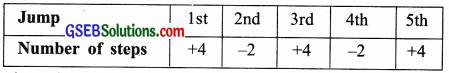∴ (b) Total number of steps =4 – 2 + 4 – 2 + 4 = 8
Also, in (a), the sum (- 8) represents going down by 8 steps.
Therefore in (b), the sum. (+ 8) will represent going up by 8 steps.